cancel
Showing results for
Did you mean:Helper I

## Distinct count how many users failed repeatedly

Hi there,

Suppose i have this table (date is in dd/mm/yyyy):

user  | date       | sales

a       | 1/1/21    | 80

a       | 1/2/21    | 110
a       | 1/3/21    | 150
b       | 1/1/21    | 75
b       | 1/2/21    | 75
c       | 1/1/21    | 30

c       | 1/2/21    | 20

c       | 1/3/21    | 100

The target sales for every month is 100. Now, we can see here for example user A failed on January, and B and C both failed on january and february.

My goal here is to count how many and who failed repeatedly, in this case is b and c, in a measure.

The result if i put date and the measure together should look like this:

date          | repeater

1/2/2021  | 2

My current DAX looks like this:

``failed count = COUNTX( FILTER( SUMMARIZE( Table, Table[user], CALCULATE( SUM(Table[sales])) < 100), Table[user])``

``previous failed count = COUNTX( FILTER( SUMMARIZE( Table, Table[user], CALCULATE( CALCULATE( SUM(Table[sales]), DATEADD(Calendar[Date], -1, MONTH)) < 100), Table[user])``

``````repeater = IF(
NOT( ISBLANK( CALCULATE([failed count], DATESMTD(Table[date]))))
&&
NOT( ISBLANK( CALCULATE([failed count], DATESMTD( DATEADD( Table[date], -1, MONTH)))))
, 1, BLANK())``````

Tried this way but it returns blank.

Any help?

6 REPLIES 6Super User

Hi,

Hope this helps.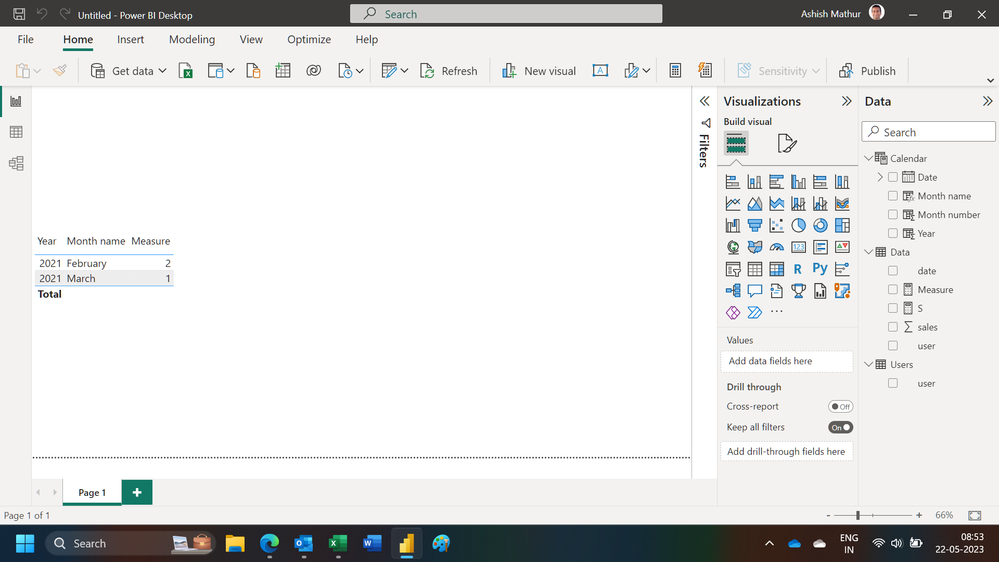Regards,
Ashish Mathur
http://www.ashishmathur.com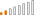Continued Contributor

Hello @aidelapplicate

The below dax would provide the desired output you are looking for. Please add a index using PQ before implementing this.

Repeater =
COUNTX(FILTER(SUMMARIZE('Table','Table'[Index],'Table'[date],'Table'[user],'Table'[sales],"Previous",CALCULATE(sum('Table'[sales]),ALL('Table'),'Table'[user]=EARLIER('Table'[user])&&('Table'[Index])=EARLIER('Table'[Index])-1),"Distinct_User_Month",CALCULATE(COUNTROWS('Table'),ALLEXCEPT('Table', 'Table'[User]),MONTH('Table'[Date]) = MONTH(EARLIER('Table'[Date])))),[Distinct_User_Month]=1&&'Table'[sales]<100&&[Previous]<100&&[Previous]<>BLANK()&&'Table'[sales]<>BLANK()),DISTINCTCOUNT('Table'[user]))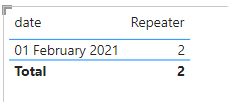Below is the dataset i used for this solution, I have taken care of same month failure as well.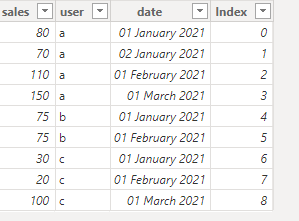If this post helps, then please consider Accept it as the solution to help the others find it more quickly.Helper I

Thank you!

I see where your are going with this, and actually really thought it would work.

Unfortunately it returns blank on my side. For your info, sales column is actually a measure too. Derivated from some simple calculation. So i made few changes in your DAX a bit like use [sales] instead of Table[sales]. Do you think this affect the result?Frequent Visitor

Hello aidelapplicate,

I think i understand your issue and have created a powerbi report based on your example data set.

I got the following results while trying to create what you described: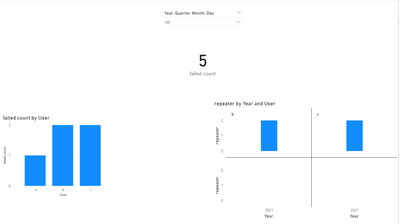I hope this is what you are looking to do.

I have created this using the following two measures:

``````failed count =
CALCULATE(
COUNT(Data[User]),
Data[sales] < 100
)``````
``````repeater =
IF(
[failed count] > 1,
CALCULATE(
COUNT(Data[User]),
Data[sales] < 100
),
BLANK()
)``````

Hope this helps.

Have a nice day!Helper I

Thank you so much for your replies.

Forgot to mention, i actually wants to find the repeater in the following month. if the user failed more than once in the same month, that doesnt count as repeater. if the user failed in month 1 and month 2, means the user is a repeater in the month 2. hope this clarifies.Frequent Visitor

I see, you can add a check to the repeater to look at the previous month like this:

``````repeater =
IF(
[failed count] > 1,
CALCULATE(
COUNT(Data[User]),
Data[sales] < 100
),
BLANK()
)``````

Hope this helps!